Matt James

•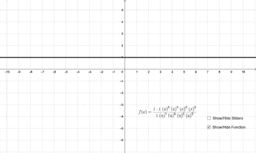Rational Function Multiplicity of Zeros, Vertical Asymptotes

Activity

Matt James

•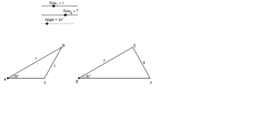4.5 SSA 2 Triangles Example

Activity

Matt James

•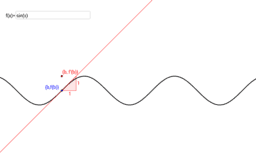Derivative of Sine

Activity

Matt James

•Exponential Derivative

Activity

Matt James

•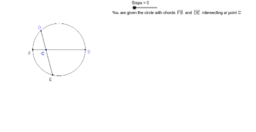Relationships of segment lengths from the intersection of secants, chords, and tangents of a circle

Activity

Matt James

•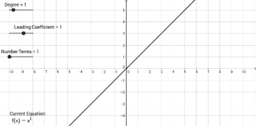Properties of Polynomial Functions

Activity

Matt James

•Pythagorean Theorem Proof

Activity

Matt James

•Pythagorean Theorem Proof

Activity

Matt James

•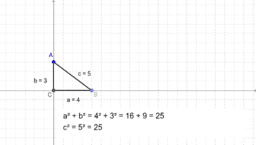Classifying Triangles from the Pythagorean Theorem

Activity

Matt James

•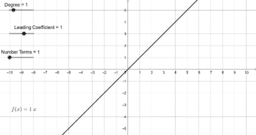Properties of Polynomial Functions

Activity

Matt James

•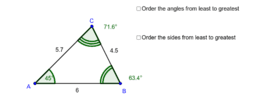Angle from Side and Side from Angle Inequalities

Activity

Matt James

•Hyperbola

Activity

Matt James

•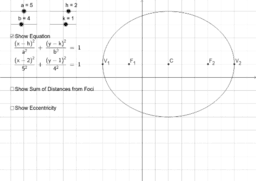Ellipse from Definition and Graphing with Transformations

Activity

Matt James

•Ellipse from Definition and Graphing with Transformations

Activity

Matt James

•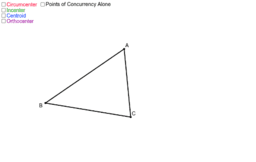Points of Concurrency (Crcmcntr, Incntr, Cntroid, Orthocntr)

Activity

Matt James

•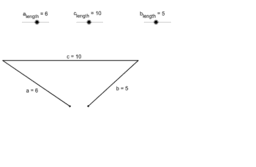Triangle Side Inequality

Activity

Matt James

•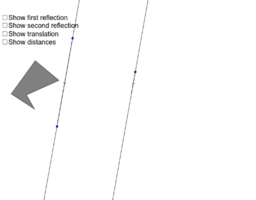Double Reflection Over Parallel Lines

Activity

Matt James

•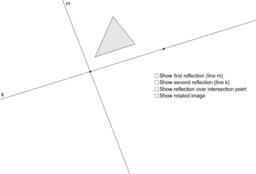Double Reflection Over Intersecting Lines

Activity

Matt James

•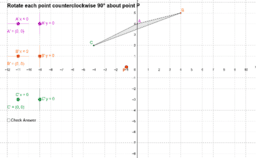Rotation of points in a triangle quiz

Activity

Matt James

•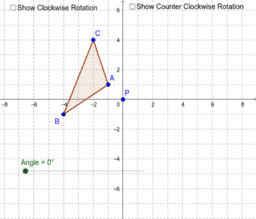Rotation

Activity

Matt James

•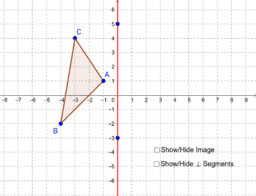Reflection

Activity

Matt James

•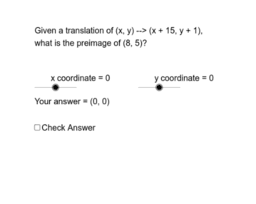Coordinate Notation Translation Quiz

Activity

Matt James

•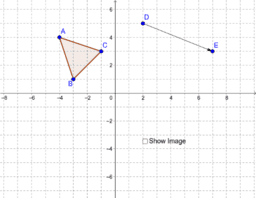Translation

Activity

Matt James

•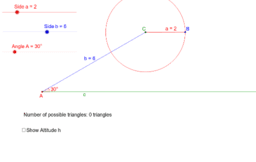Activity

Matt James